# Insert File Name – Excel Formula

This tutorial will teach you how to get a file name using a formula in Excel.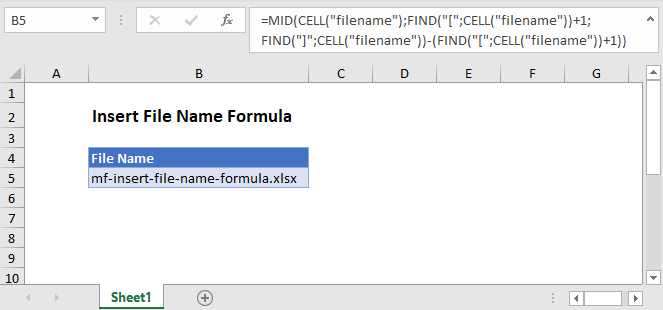## Get File Name

In Excel there isn’t a function to get the file name directly. However, the CELL Function will return the file path, name, and sheet. Using the text functions FIND and MID, you can extract just the file name.

``````=MID(CELL("filename"),FIND("[",CELL("filename"))+1, FIND("]",CELL("filename"))-
(FIND("[",CELL("filename"))+1))``````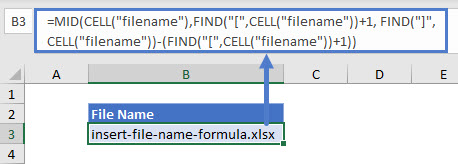Let’s step through the formula.

## File Name, Path, and Worksheet

We use the CELL Function to return the file path, name, and sheet by entering “filename” as the info type.

``=CELL(“filename”)``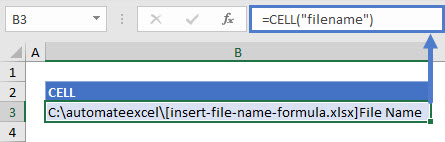### FIND the File Name Position

As shown above, the CELL Function returns the file path, name, and worksheet. We don’t need the path and the worksheet name, so we use the FIND Function to determine the position of the character immediately before (“[“) and after (“]”) the file name.

This first formula will look for “[“. We add one to the first position to start after the first square bracket (“[“).

``=FIND("[",CELL("filename"))+1``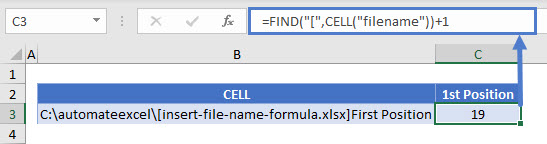Next we will calculate the position of the “]” to determine where the file name ends.

``=FIND("]",CELL("filename"))``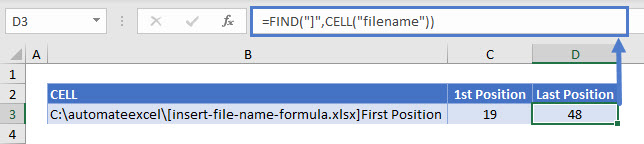Take the difference to find the length of the file name: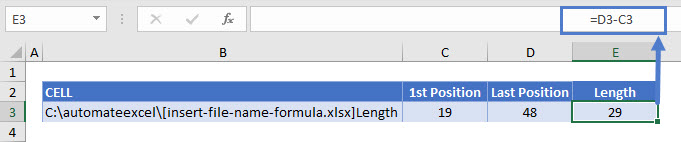## MID Function

Now we have the starting position and the length of the file name. We plug these results into the MID function to extract the file name from the file path, name, and worksheet string.

``=MID(B3,C3,E3))``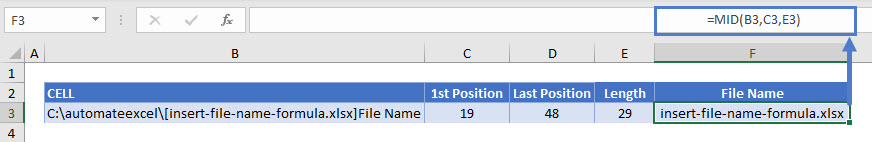Combing these steps into a single formula, we get:

``````=MID(CELL("filename"),FIND("[",CELL("filename"))+1, FIND("]",CELL("filename"))
-(FIND("[",CELL("filename"))+1))``````### Excel Practice Worksheet

Practice Excel functions and formulas with our 100% free practice worksheets!